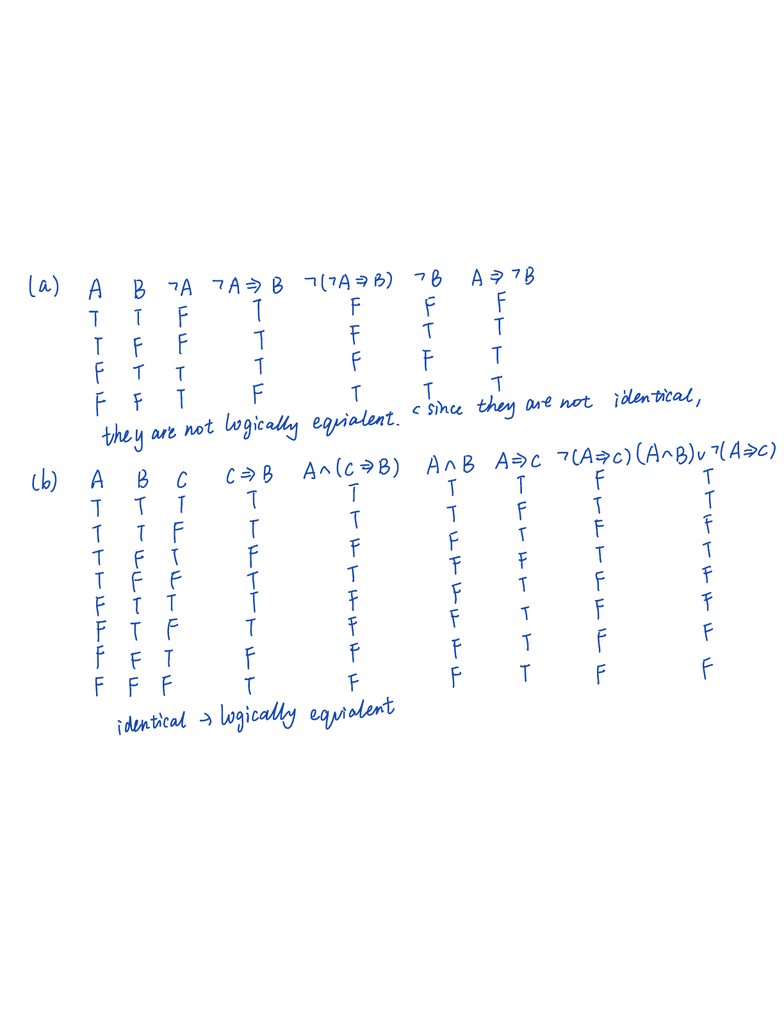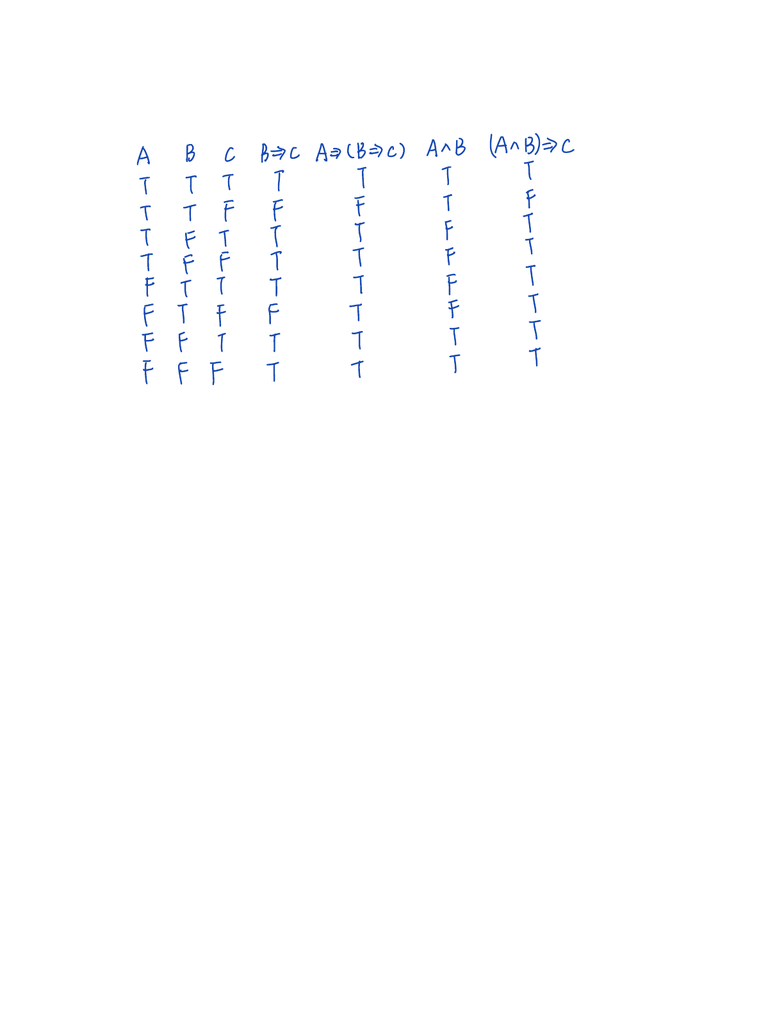Study Guides (390,000)
CA (150,000)
UW (7,000)
MATH (600)
MATH135 (100)
Midterm

# MATH135 Midterm: A01Exam

Department
Mathematics
Course Code
MATH135
Professor
Alan Thompson
Study Guide
Midterm

This preview shows pages 1-2. to view the full 7 pages of the document.MATH 135 Spring 2019: Assignment 1
Due at 8:30a.m. on Friday, May 17, 2019
1. Let A,B,andCbe statement variables.
(a) Prove that ¬(¬A=)B)6⌘ (A=)¬B).
(b) Use a truth table to prove that
A^(C=)B)(A^B)_¬(A=)C).
aABTA TA B717A B713 A713
TTFTFFF
TFFTFTT
FTTTFFT
FFTFTTT
theyare notlogically equialene sincetheyare not identical
cBAacc BAaB AcVA cAaB uYA c
bABCyTTET
TTTTTFTT
TTFTTftFF
TFTFFpFTT
TFFTTTFF
FTTTFFTFF
FTFTFFyfF
FFTFFF
FFFTFFTFF
identical logically equialent

Only pages 1-2 are available for preview. Some parts have been intentionally blurred.2. Let A,B,andCbe statement variables. Prove that A=)(B=)C)islogically
equivalent to (A^B)=)C.
ABCBcA cB cAaB AaB c
TTTTTTT
TTFFFTF
TFTTTFI
TFFTTF
FTTTTFT
FTFFTFT
FFTTTTT
FFFTTTT